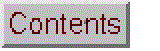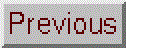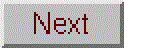# Multiwavelength Spectrophotometric Analysis by Classical Least Squares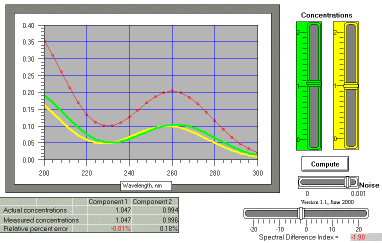WingZ version (2 or 3 components) CLS1: two-component model with 26 wavelengths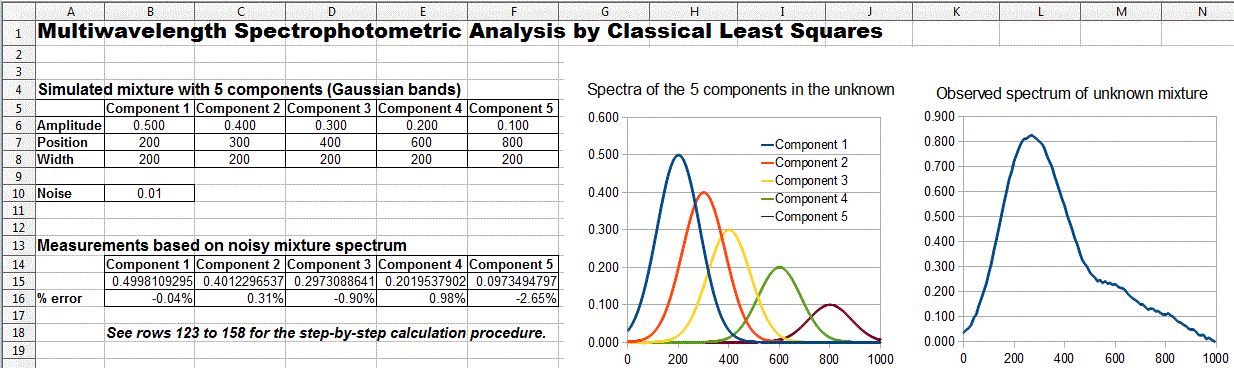Excel and OpenOffice Calc versions (5 components) Five components with 100 wavelengths

This is a simulation of UV multicomponent absorption spectroscopy of a mixture consisting of two or three absorbing components whose absorption spectra are known (but whose concentrations in the mixture are unknown and are to be determined). The simulation allows you to create simulated mixtures of the components and then to analyze the mixture using the observed total absorption spectrum and the absorptivities of the components, using the "classical least-squares" (CLS) method. Realistic amounts of random noise can be added to the "observed" mixture spectrum in order to demonstrate the precision obtainable with this method.

Operating instructions (Excel and OpenOffice Calc versions)
In these versions, the simulated mixture is defined by the peak amplitudes, positions, and widths of the 5 components that you type into the table in columns A-F, rows 6-8. The noise level is determined by typing into Noise, in column B, row 10. The spreadsheet automatically plots the spectra of the five components (each component with a different color) and the spectrum of the mixture with noise added, then automatically calculates the amplitude (concentrations) of each component in the simulated mixture and the percent error (relative difference between the true and measured amplitudes). You can test the limits of this method by placing the peak positions of the components closer together, increasing their widths, or increasing the noise, thereby creating a more challenging problem to solve. If you are curious how this calculation is performed, the details are laid out step-by-step in rows 123 to 158.

Operating instructions (WingZ version)
The relative concentrations of the components are controlled by the colored sliders on the right. The green slider controls the concentration of component 1, the yellow slider controls the concentration of component 2, and, for the three-component version, the blue slider controls the concentration of component 3 (or of the background absorption, in CLS1b and CLS4). The spectra of the components separately are shown on the graph as the solid lines matching the color of the slider that controls it. These concentrations are the "known unknowns" for the simulation. Every time you change these sliders, you are simulating a different sample mixture.

The small "noise" slider below the compute button determines the amount of total absorbance noise. Its range is from zero (left-most position) to 0.001 (right-most setting). This amount of random noise is added to the mixture spectrum to approximate the effect of random instrumental noise (flicker, photon, and detector dark noise). Without this noise, the results of the simulation would be unrealistically "perfect". Every time you change this slider, you are simulating the effect of a different instrument (with more or less random instrumental noise).

The Spectral Difference Index slider controls the similarity of the shapes of the component spectra. When this is zero, there is no difference between the shapes of the components. This control basically controls the overlap between the spectra. The purpose of this control is to allow you to simulate different mixture systems of varying degrees of similarity so you can determine how much difference between spectra is necessary for good measurement. Every time you change these sliders, you are simulating a different chemical system (different compounds).

When you click on the Compute button, a classical least squares computation is performed, based on the simulated measured mixture spectrum with noise added (red dots) and using the known shapes of the component spectra. That is, it attempts to calculate the component concentrations from the mixture spectrum. The results of this calculation are shown in the table below the graph as the "Measured concentrations". The "Actual" concentrations (that you set using the sliders) is also shown, as well as the relative percent difference between them. Every time you click on this button, you are simulating another reading of the mixture spectrum and a repeat of the CLS calculation. The results will be slightly different each time because of the noise.

Assumptions: The system obeys Beer's Law and absorbances are additive. Except for CLS1b, the only absorption in the mixture arises from the known components. Except for CLS1b and CLS4, background absorption (baseline shifts, irrelevant absorption, cell reflections, and light scattering) is assumed to be zero. The absorptivities of the components are assumed to be the same in the mixture as in the standard solutions from with the absorptivities are measured, and the absorptivities are measured at sufficiently high concentrations so that the random noise in the absorptivity spectra is negligible; thus random noise effects only the mixture spectrum.

Note: The classical least squares method works properly only if the absorbance is directly proportional to concentration according to the Beer-Lambert Law.  If non-linearity occurs because of polychromaticity or stray light, a more advanced computational method, the transmission fitting method, may be employed.

CLS4 is like CLS3, except that the third component is a flat background absorption. Download link: CLS4.wkz.
Wingz player application and basic set of simulation modules, for windows PCs or Macintosh

RegressionDemo.xls and RegressionDemo.ods (for Excel and OpenOffice Calc, respectively)

Other related simulations:
Curve Fitting B: Multicomponent Spectroscopy
Signal-to-noise ratio of absorption spectrophotometry
Transmission fitting method (a multicomponent method that does not require Beer's Law linearity)

Instructor's note on the different versions.

Some mathematical background on the classical least squares method applied to multicomponent spectroscopy can be found in Curve fitting B: Multicomponent Spectroscopy.

CLS1 can be compared to CLS2 to demonstrate the slightly increased precision resulting from the use of a greater number of wavelengths (better averaging out of the random noise). CLS1 can be compared to CLS3 to demonstrate the additional computations required to handle one more component (and the increasing computation time, noticeable only on slow computers). CLS1 can be compared to CLS1b to demonstrate what happens if there is absorption occurring in the mixture solution that is not included in the model (namely, gross errors). CLS1b can be compared to CLS4 to demonstrate how the error resulting from a flat background absorption can be corrected by including the background absorption as a third component and performing a 3-component computation.

These models can be operated using only the mouse-activated on-screen sliders, for use in a lecture-demonstration environment with a computer video projection system, where it is difficult to use the keyboard data entry in a darkened room. However, the concentrations of the components can also be typed into the table below the graph (in the row labeled "Actual concentrations") in order to specify concentrations beyond the limits of the sliders.

Cell definitions and equations (for CLS1):
Inputs:

Concentration of component 1, Conc1: cell D25 (controlled by the green slider)
Concentration of component 2, Conc2: cell E25 (controlled by the yellow slider)
Random noise amplitude, N: cell B29 (controlled by the "Noise" slider)
Spectral difference index, D: cell J28 (controlled by the "Spectral difference" slider)

Spectral width index, W: cell A29
Absorption weighting factor for component 1, weight1: cell D29
Absorption weighting factor for component 2, weight2: cell F29

Spectral array calculations (for CLS1):

Wavelength: A30..A55 (200 to 300 nm in 0.4 nm steps (0.2 nm steps for CLS2)
Absorptivity of component 1: D30..D55 = weight1 * (2*exp(-(Wavelength-190-D) * (Wavelength-190-D)/W)+
exp(-(Wavelength-260-D)*(Wavelength-260-D)/W))
Absorptivity of component 2: F30..F55 = weight2 * (2*exp(-(Wavelength-190+D) * (Wavelength-190+D)/W)+
exp(-(Wavelength-260+D)*(Wavelength-260+D)/W))
Random noise at each wavelength: G30..G55 = N * (rand()-rand())
"Observed" mixture spectrum: B30..B55 = Conc1 * (D30..D55)+Conc2*(F30..F55)+(G30..G55)

The spectrum of component 1 consists of two Gaussian bands, one at 190+D nm
with amplitude 2*weight1, and the other at 260+D nm, with amplitude weight1, and
the spectrum of component 2 consists of two Gaussian bands, one at 190-D nm
with amplitude 2*weight2, and the other at 260-D nm, with amplitude weight2,
where D is the spectral difference index. If D is zero, the two spectra are
identical in shape. The width of the component bands of both spectra is
controlled by W in cell A29 (if you make this larger, the bands will be
broader). The default value for W is 700. The default value for weight1 and
weight2 is 0.1 (cells D29 and F29, respectively).

The "observed" mixture spectrum is simulated by adding the absorbances (concentration times absorptivity) of the two components and then adding random noise (G30..G55) whose amplitude is controlled by N (the "noise" slider).

Classical Least Squares matrix calculations (for CLS1):

E is the 2 X 25 matrix of absorptivities of the two components at each wavelength (A107..B132,
formed by fusing D30..D55 and F30..F55).
A is the 1 X 25 matrix of observed absorbances of the mixture at each wavelength
(B30..B55, mirrored in N24..N55).
The 1 X 2 matrix of calculated concentrations (F119..F120) is given by A(ETE)-1ET.
These results are mirrored in the display area (D26 and E26).

The Compute button activates a "script" that automates the computation by utilizing
the built-in matrix computation operations of the spreadsheet which are under the

Matrix item in the Sheet menu. To see how the computations are performed,
scroll the spreadsheet window to the right (click on the right-pointing arrow on the
scroll bar at the bottom of the window) until you see the "Step-by-step calculation
procedure", which shows the actual matrices as grey blocks, each labeled, for each step
of the calculation:
```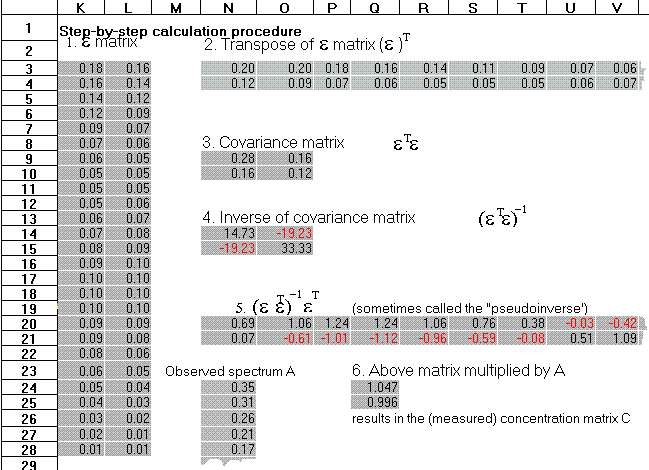Pull down the Sheet menu and the Matrix sub-menu and notice the Transpose, Invert, and Multiply operations which are needed for the CLS calculation.

"Compute" button script that performs the Classical Least Squares matrix calculations):repaint offselect range A107..B132Copyselect range C107paste valuesTransposeselect range C107..AB108select more range A107..B132select more range F110Multiplyselect range F110..G111select more range F113Invertselect range F113..G114select more range C107..AB108select more range F116Multiplyselect range F116..AE117select more range B30..B55select more range F119Multiplyrecalcrepaint onselect range A1```

### Student Assignment

Download the WingZ player application as described above. Launch wingz.exe, then open CLS1.wkz.

1. Set the Spectral Difference Index slider to -20 and both component concentrations to 1.0. Would it have been possible to measure either of these components by a simple single-wavelength calibration? That is, are there wavelengths where only one of the components absorb?

2. How does the noise level effect the average accuracy of measurement? What happens if you to the error if you make the noise zero?

3. Does varying the concentration of component 1 while holding component 2 constant cause a systematic change in the measured concentration of component 2?

4. What is the smallest concentration of component 1 you can measure with a relative accuracy of 1% when the concentration of component 2 is held at 2? How does the noise effect this?

5. Set both concentrations to 1 and the noise to 0.001 (maximum). Vary the Spectral Difference Index slider to make the two spectra more similar. How similar can the be and still be measured to a relative accuracy of 1% . How does the noise effect this?

6. Set the noise to zero (left-most position) and force the Spectral Difference Index to exactly zero by typing a 0 into the gray cell to the right of the = sign in "Spectral Difference Index =". This simulates two components with absolutely identical spectra, but with zero noise. What happens when you click on the Compute button. Why?

7. To see how the computations are performed, scroll the window to the right (click on the right-pointing arrow on the scroll bar at the bottom of the window) until you see the "Step-by-step calculation procedure", which shows the actual matrices as gray blocks, each labeled, for each step of the calculation. Which matrices change when you recalculate after changing the concentration sliders? The noise slider? The Spectral Difference Index slider? Why?

8. Open up the file called "CLS2". This is similar to CLS1 except that readings are taken every 2 nm, for a total of 51 wavelengths (w =51). Can you expect any differences in calculation speed or accuracy? Do you observe any difference? Explain. (Hint: There is a difference, theoretically, but it's small and you may miss it).

[RETURN] to the Spreadsheet Simulations Index.

[RETURN] to Chem 623 WingZ page.

(c) 1991, 2015. This page is part of Interactive Computer Models for Analytical Chemistry Instruction, created and maintained by Prof. Tom O'Haver , Professor Emeritus, The University of Maryland at College Park. Comments, suggestions and questions should be directed to Prof. O'Haver at toh@umd.edu. Number of unique visits since May 17, 2008: